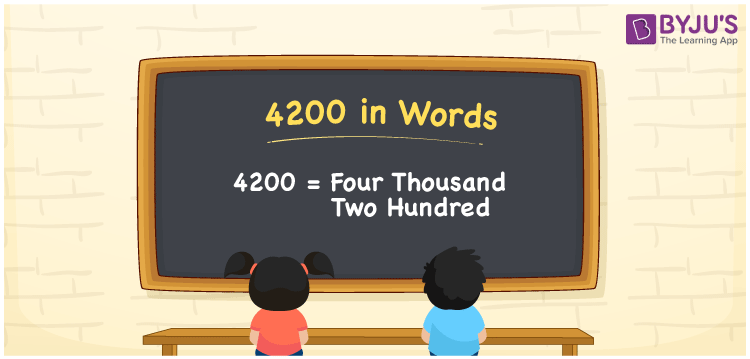# 4200 in Words

4200 in words can be written as Four Thousand Two Hundred. In this article, you will get to know the importance of numericals and counting. For example, if you buy a helmet for Rs. 4200, then you can say that “I bought a helmet for Four Thousand Two Hundred Rupees”. Learn how to write numbers in words from the table given below. So, 4200 can be read as “Four Thousand Two Hundred” in words.

 4200 in words Four Thousand Two Hundred Four Thousand Two Hundred in Numbers 4200

## 4200 in English Words## How to Write 4200 in Words?

The place value chart of 4200 can be learnt with ease from the table. There are four digits in the number 4200 and the place value of each digit is shown below.

 Thousands Hundreds Tens Ones 4 2 0 0

4200 in expanded form is:

4 x Thousand + 2 × Hundred + 0 × Ten + 0 × One

= 4 x 1000 + 2 × 100 + 0 × 10 + 0 × 1

= 4000 + 200

= 4200

= Four Thousand Two Hundred

Hence, 4200 in words is written as Four Thousand Two Hundred.

4200 is a natural number that precedes 4201 and succeeds 4199.

4200 in words – Four Thousand Two Hundred

Is 4200 an odd number? – No

Is 4200 an even number? – Yes

Is 4200 a perfect square number? – No

Is 4200 a perfect cube number? – No

Is 4200 a prime number? – No

Is 4200 a composite number? – Yes

## Frequently Asked Questions on 4200 in Words

Q1

### How do you write 4200 in words?

4200 can be written as “Four Thousand Two Hundred” in words.
Q2

### What is the value of 4500 – 300?

4500 – 300 is 4200. It is Four Thousand Two Hundred in words.
Q3

### How can Four Thousand Two Hundred be written in numbers?

Four Thousand Two Hundred can be written in numbers as 4200.